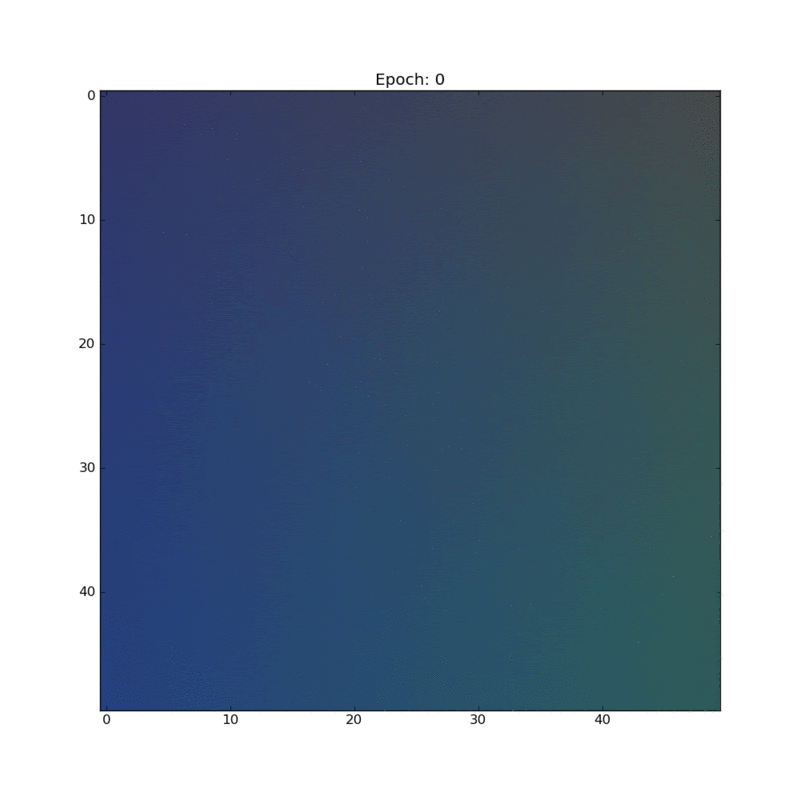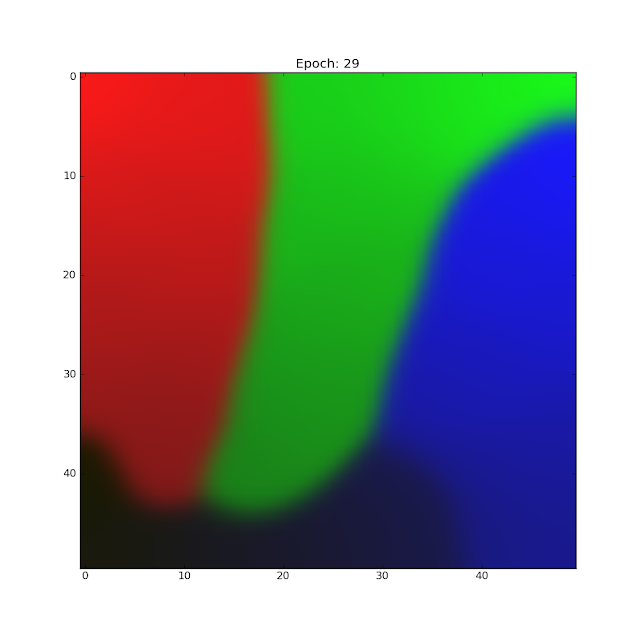## Wednesday, 30 March 2016

### Unsupervised Learning: Self Organizing Maps

Most machine learning techniques like logistic regression, linear regression, and neural networks do something that is called supervised learning. This means that we need to supply examples of "correct" data to the machine learning algorithm for it to learn the patterns in the data. The goal of unsupervised learning is to automatically find clusters of similar data in a huge unlabeled set of data. Humans can do this easily with some types of data. If you saw a 2D scatter plot of points you'd easily be able to identify clusters in the data by visual examination. I've always considered unsupervised learning cooler than supervised mostly because it feels like that's what a general AI should be able to do.

Self organizing maps (SOMs) are one technique used to find these clusters in the data. A self organizing map looks a little similar to a neural network at first. But the kind of operation that it does is very different. An SOM has a lattice of nodes. This lattice is usually one dimensional(arranged in a linear array) or 2 dimensional (arranged in a matrix). As the self organizing map is trained, the lattice of will be partitioned into separate classes. Since one of the main uses of self organizing maps is visualizing higher dimensional data, the lattice is is rarely more than two dimensions wide. The clusters in the higher dimensional data can be visualized on the 2D lattice.  Associated with each of the nodes in the lattice is a weight vector that has the same dimension as the input data vectors. To train the self organizing map we take each element in the data set and find the node with the weight that's closest to the element (Using the euclidean distance as the measure of closeness. Other measures can be used too.). Then we define a neighbourhood of nodes that are 'close' to this best matching unit and we adjust the weights of this entire neighbourhood of weights to be a little closer to the value of the element from the dataset. How much the weights are adjusted depends on the neighbour falloff function and on the learning rate. This can be expressed in one simple equation.
$$W_{uv}(t+1) = W_{uv}(t) + \Omega (u,v,t) \alpha (t)[x_n - W_{uv}(t)]$$
In each iteration, the learning rate and the size of the neighbourhood function is reduced by a small amount. The neighbourhood size is initially set to a large value. This allows the algorithm to initially affect the weights on a global scale and then over time adjust smaller and smaller regions of the map to train it.

In my implementation I used a Gaussian neighbourhood function whose variance decayed exponentially. The learning rate started off at 0.1 and decayed exponentially from there.

To check my implementation, I tested something that is a common use for SOMs. Clustering colors in an RGB color space. Colors are easy to visualize and I thought it would make a good demo. I first generated a random data set of bluish, reddish, greenish and dark bluish colors.A random sampling of 4 different colors for the dataset.
I initialized the weights randomly and started the training. For this particular experiment I used a 2D 50x50 lattice of nodes. Since each node has a weight that is a 3 dimensional vector that represents a color, we can visualize the SOM node lattice as an RGB image. Stacking together the weight visualization after each epoch (One pass through the training set) of training gave me this really beautiful looking animation! :DAnimation that shows the self organizing map after each epoch of training.
You can clearly see the different clusters of color slowly resolving as the training happens. The initial changes are more global due to the large neighbourhood. But as the training progresses the adjustments to the weights become more and more local. There is also a very nice separation of the different colors that were present in the first image. You can see that the brighter colors seem to be congregating towards the top of the image and the darker colors towards the bottom. The darkest blue (maybe even a little black) ended up in the bottom left corner surrounded by the darker hues of red, green and blue.The final map after 30 epochs of training.

## Tuesday, 22 March 2016

### Machine Learning Part 2: Implementing Multi Class Logistic Regression

In my last post on machine learning I talked about how to implement simple linear regression in Python. This time I am going to implement logistic regression. This technique is used to classify input data into one of several classes.

First let's take a look at two class regression.

Let's have a set of input vectors $\{x_1, x_2, ... , x_N\}$ and a set of targets $\{t_1, t_2, t_3, ..., t_N\}$ as our training data. A logistic regression classifier defines a model $y(x,w) = h(w^Tx)$. The function $h(a)$ is a logistic sigmoid function or a "squashing" function that takes the output of the linear function $w^Tx$ and sqeezes it into a range of values from 0 to 1. Using the logistic sigmoid function allows us to interpret the output of the classifier as the probability that the input belongs to class 1 under certain assumptions.

So just like in linear regression we need to define cost function in terms of the parameters so that we have a measure of the performance of the model and a way to train the model. In the case of logistic regression there is a cost function - that is very effective and commonly used - called the cross entropy cost function. This cost function is again selected based on a probabilistic interpretation of the classifier.

$$J = -\sum_{n=1}^{N}t_n ln(y(x_n) + (1-t_n)ln(1-y(x_n))$$

The gradient of this cost function is surprisingly the same as the gradient of the square error cost function.

$$\frac{\partial J}{\partial W} = \sum_{n=1}^{N}(y(x_n) - t_n)x_n$$

The weights of the model can be adjusted using the gradient descent algorithm just like in linear regression. The classifier will generate a straight that will separate the two classes.

Multiclass logistic regression can be done using many logistic regression units if the goal is to perform multiple binary classifications. However, if you have a vector that needs to be assigned to one of K classes, the procedure is slightly different. Multiclass regression is performed using the model $y(x) = softmax(W^Tx + \vec{b})$ where x is the input vector, W is a matrix with K columns where each column is a parameter vector, $\vec{b}$ is a vector of biases and the softmax function is defined as follows.

$$softmax(a_j) = \frac{e^{a_j}}{\sum_{k=1}^{K}e^{a_k}}$$

Each of the targets to train the classifier will be an array of size K. If an input belongs to class k, the kth position of the array will be a one. The rest of the elements in the array will be zero. This is called 'one-hot encoding'. It is easy to see from the definition of the softmax function that the output of the classifier will be an array of values  that are between zero and one and that the sum of all the elements in the output array will be 1. This means that we can interpret the output array as a probability distribution that tells you the probabilities that the input vector belongs to each of the K classes.

Like in two class classification, the input data (with N vectors and dimension M) set can be arranged in a NxM array $X$ where each row is an input vector. The corresponding targets can be arranged in a matrix T that has N rows and K columns. If the input vector $x_n$ belongs to class K the element $T_{nk}$ will be one. The rest of the elements of the matrix will be zero.

The cost function is the same cross entropy function extended to work with the matrix T.
$$J = -\sum_{n=1}^{N}\sum_{k=1}^{K}T_{nk}ln(y_k(x_n))$$
However, since we are using a softmax function instead of the logistic sigmoid function, the gradient of the cost function will be different. The derivation to obtain expression for the gradient gets very hairy but the expression for the gradient is surprisingly simple.

$$\frac{\partial J}{\partial W_{uv}} = \sum_{n=1}^{N}X^T_{vn}(t_{nu} - y_{nu})$$

Once we have this gradient, gradient descent can be performed on the parameters in the same way. I gathered all the vectorized implementations of the equations I've mentioned into a classifier class in Python for ease of usage.

To test the classifier I created a scatter of normally distributed points centred around different means on a 2D plane and applied the classifier. With a little bit of help from stackoverflow I was able to figure out how to color the regions of the plot according to the trained classifier.This is a scatter plot of the unclassified data. The points were generated using multivariate normal distributions using different means.This is a plot of the error or the cost over time as the gradient descent algorithm runs.A plot of the decision regions learned by the classifier. If a region is shaded a particular color, that means that the classifier identifies all the points in that region as belonging to the same class.